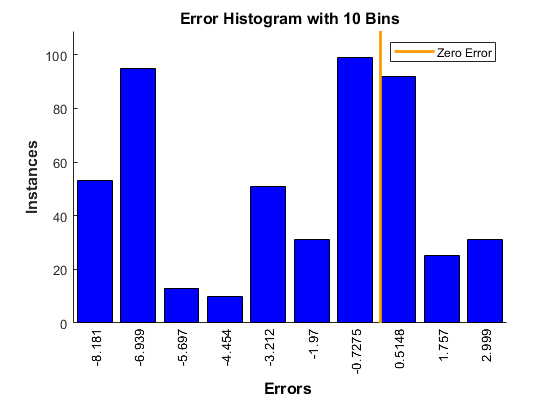# Plot Error Histogram for a Neural Network

This example shows how to visualize errors between target values and predicted values after training a feedforward neural network.

### Read Data from the Weather Station ThingSpeak™ Channel

ThingSpeak channel 12397 contains data from the MathWorks® weather station, located in Natick, Massachusetts. The data is collected once every minute. Fields 2, 3, 4, and 6 contain wind speed (mph), relative humidity, temperature (F), and atmospheric pressure (inHg) data, respectively. Read the data from channel 12397 using the `thingSpeakRead` function.

```data = thingSpeakRead(12397,'Fields',[2 3 4 6],'Numpoints',500,'outputFormat','table'); ```

Assign input variables, and calculate dew point from temperature and relative humidity to use as the target. Convert temperature from Fahrenheit to Celsius, and specify the constants for water vapor (b) and barometric pressure (c). Calculate the intermediate value 'gamma', and assign target values for the network.

```inputs = [data.Humidity'; data.TemperatureF'; data.PressureHg'; data.WindSpeedmph']; tempC = (5/9)*(data.TemperatureF-32); b = 17.62; c = 243.5; gamma = log(data.Humidity/100) + b*tempC ./ (c+tempC); dewPointC = c*gamma ./ (b-gamma); dewPointF = (dewPointC*1.8) + 32; targets = dewPointF'; ```

### Create and Train the Two-Layer Feedforward Network

Use the `feedforwardnet` function to create a two-layer feedforward network. The network has one hidden layer with 10 neurons and an output layer. Use the `train` function to train the feedforward network using the inputs.

```net = feedforwardnet(10); [net,tr] = train(net,inputs,targets); ```

### Use the Trained Model to Predict Data

After the network is trained and validated, you can use the network object to calculate the network response to any input, in this case the dew point for the fifth input data point.

```outputs = net(inputs(:,5)) ```
```outputs = 22.8618 ```

### Plot the Error Histogram

Compute the error values as the difference between target values and predicted values.

```error = targets - outputs; number_of_bins = 10; ploterrhist(error,'bins',number_of_bins); ```The plot shows an error histogram with 10 bins.